# Answered! Find an optimal solution to the following transportation problem. The total cost of the optimal solution S From…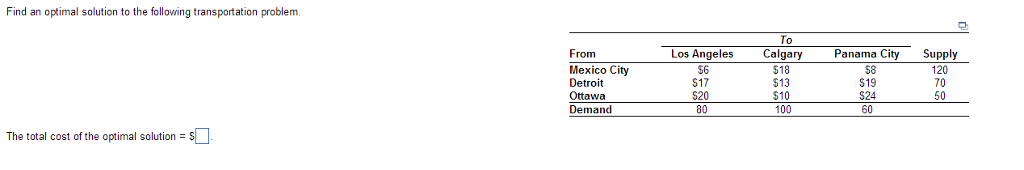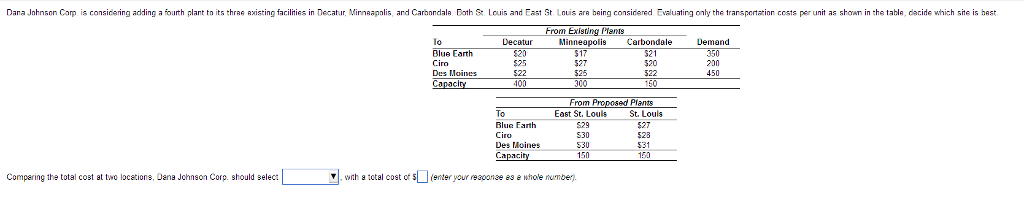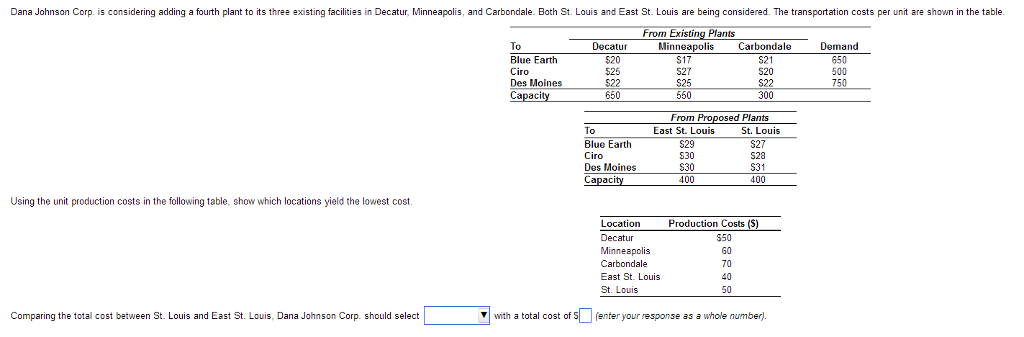Find an optimal solution to the following transportation problem. The total cost of the optimal solution S From Mexico City Detroit Ottawa Demand Los Angeles \$6 \$17 \$200 80 To Calgary \$18 \$13 100 Panama City Supply 120 S19 60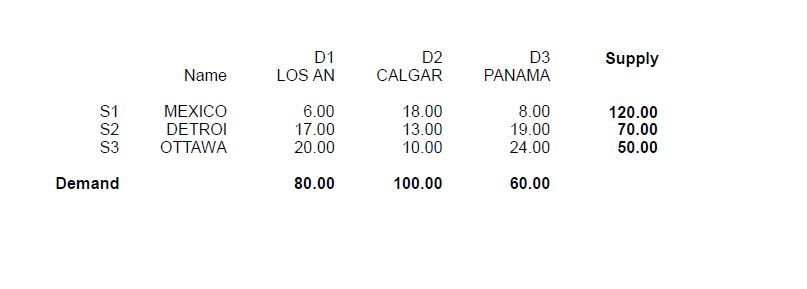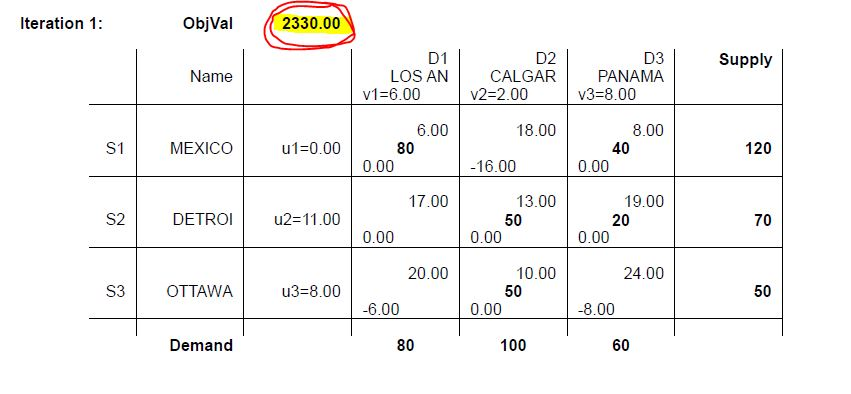TOTAL COST=80*6+40*8+50*13+20*19+50*10=\$2330